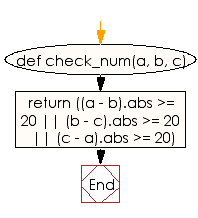﻿ Ruby Basic exercises: Check three given integers and return true if one of them is 20 or more less than one of the others - w3resource# Ruby Basic Exercises: Check three given integers and return true if one of them is 20 or more less than one of the others

## Ruby Basic: Exercise-49 with Solution

Write a Ruby program to check three given integers and return true if one of them is 20 or more less than one of the others.

Ruby Code:

``````def check_num(a, b, c)
return ((a - b).abs >= 20 || (b - c).abs >= 20 || (c - a).abs >= 20)
end

print check_num(9, 12, 22),"\n"
print check_num(112, 202, 20),"\n"
print check_num(102, 203, 405)
``````

Output:

```false
true
true
```

Flowchart:Ruby Code Editor: